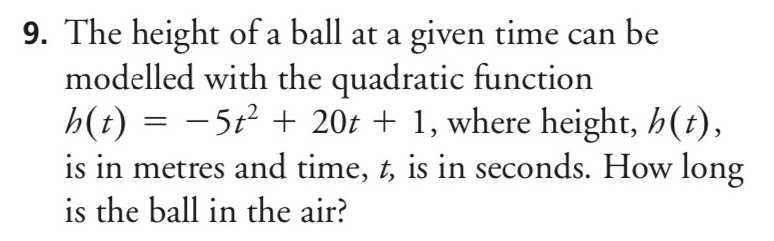### ¿Todavía tienes preguntas de matemáticas?

Pregunte a nuestros tutores expertos
Algebra
Pregunta9. The height of a ball at a given time can be modelled with the quadratic function

$$h ( t ) = - 5 t ^ { 2 } + 20 t + 1$$ , where height, $$h ( t )$$ , is in metres and time, $$t$$ , is in seconds. How long is the ball in the air?

$$t = 2 + \sqrt { \frac { 21 } { 5 } }$$